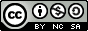## Wednesday, July 16, 2008

### Perl and Bash Versions Of Binary To Decimal Conversion Script

Hey There,

As promised, in yesterday's post on converting binary values to decimal in C, today we're going to follow up with straight-up ports to Perl and shell. Actually, they'll be slightly different. If you check yesterday's post, for some reason I left in a variable called "difference" which was a part of the code I mentioned that I had originally written to pad 0's on the left hand side of a binary number less than 8 digits. Actually, it was noticed by an LXer, too, as well as the fact that I didn't specifically initalize "char binnumber" with a value. Whoops :) Special thanks to friends who pointed out that someone had commented on that. Not enough hours in the day to check everywhere. Thanks to the folks at LXer.com for doubling my virtual shame ;) Just kidding.

Anyway, I've mulled it over and I've decided to leave the original post with the error even though it could be fixed with a simple edit. I'm as fallible as the next guy (denying it would be pompous folly) and if I spent the rest of my life doing nothing but cleaning up my mistakes I'd never learn from them or (even worse) miss out on most of the good things life has coming around the bend. It can be a chore just thinking of something to write about once today; writing quick programs can be a real sleep-stealer. But I'm going waaaay off-topic.

Both of today's scripts work exactly as they did in yesterday's post (with the bonus that these programs will know whether your variables are integers or characters just by defining them (No initialization necessary). Please check out that conversion program post for specifics on how the program works, it's purposefully imposed limitations, etc.

Both scripts (Perl and shell -- and I think this is the first time I've ever thrown two scripts into one post, so apologies if the Blogspot code formatting is worse than usual ;) run just like yesterdays code, except I've named them differently. You can, of course, change the names to whatever you want:

host # ./bin2dec.pl

or

host # ./bin2dec.sh

and both produce output that's exactly the same:

host # \$ ./bin2dec.pl

8 Digit Binary Number?
10010010

Binary 10010010 equals Decimal 146!

We're not going into an explanation of porting at this point (we're following that on a separate path in our posts on porting between Perl, shell and Awk), but you should be able to see a lot of similarities between the code.

Note that, for today, the Perl script will come first and then the shell script. They should be easy to discern. We'll see... this is my first try at doing a double-script ending. Maybe one day they'll let me do attachments (If the GoogleBot can hear me, I'll gladly pay for that :)

Cheers,`#!/usr/bin/perl# bin2dec.pl - Convert 8 digit binary numbers# to decimal numbers## 2008 - Mike Golvach - eggi@comcast.net## Creative Commons Attribution-Noncommercial-Share Alike 3.0 United States License\$total = 0;print "\n8 Digit Binary Number?\n";chomp(\$binnumber = (<STDIN>));@binnumber = split(//, \$binnumber);\$length = @binnumber;if ( \$binnumber == 1 ) { \$total += 1;}if ( \$binnumber == 1 ) { \$total += 2;}if ( \$binnumber == 1 ) { \$total += 4;}if ( \$binnumber == 1 ) { \$total += 8;}if ( \$binnumber == 1 ) { \$total += 16;}if ( \$binnumber == 1 ) { \$total += 32;}if ( \$binnumber == 1 ) { \$total += 64;}if ( \$binnumber == 1 ) { \$total += 128;}print "\nBinary \$binnumber equals Decimal \$total!\n";exit(0);`
`#!/bin/bash# bin2dec.sh - Convert 8 digit binary numbers# to decimal numbers## 2008 - Mike Golvach - eggi@comcast.net## Creative Commons Attribution-Noncommercial-Share Alike 3.0 United States Licensetotal=0echo -e "\n8 Digit Binary Number?\n"read numberlength=\${#number}set -a binnumberfor x in \$(seq 0 \$((\${#number} - 1)))do binnumber[\$x]=\${number:\$x:1}doneif [ \${binnumber} -eq 1 ]then total=\$((total + 1))fiif [ \${binnumber} -eq 1 ]then total=\$((total + 2))fiif [ \${binnumber} -eq 1 ]then total=\$((total + 4))fiif [ \${binnumber} -eq 1 ]then total=\$((total + 8))fiif [ \${binnumber} -eq 1 ]then total=\$((total + 16))fiif [ \${binnumber} -eq 1 ]then total=\$((total + 32))fiif [ \${binnumber} -eq 1 ]then total=\$((total + 64))fiif [ \${binnumber} -eq 1 ]then total=\$((total + 128))fiecho -e "\nBinary \$number equals Decimal \$total!\n"exit 0`